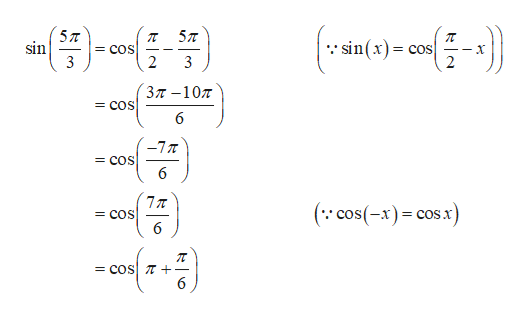# The question asks for the exact value of the trigonometric function at the given real number: sin5pi/3  I answered -(sqrt)3/2, but I want to double check this answer...

Question
29 views

The question asks for the exact value of the trigonometric function at the given real number:

sin5pi/3  I answered -(sqrt)3/2, but I want to double check this answer...

check_circle

Step 1

Obtain the exact value of the trigonometric fu...help_outlineImage Transcriptionclose5л 57T sin(x)= sin = COS = COS 3 2 37-10 Зл —107 = COS 6 -7T = COS 6 77T = COS 6 (: cos(-x) cosx = COS = COS + fullscreen

### Want to see the full answer?

See Solution

#### Want to see this answer and more?

Solutions are written by subject experts who are available 24/7. Questions are typically answered within 1 hour.*

See Solution
*Response times may vary by subject and question.
Tagged in

### Trigonometric Ratios